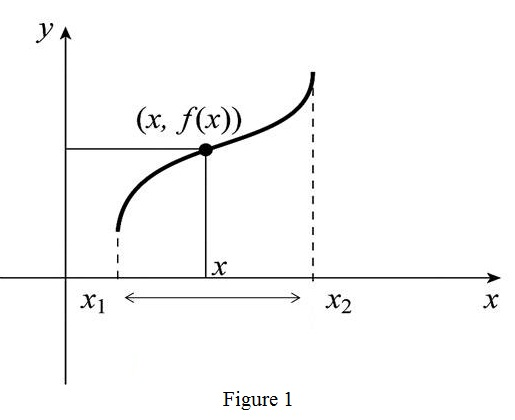# The terms function, domain of a function and range of a function.### Single Variable Calculus: Concepts...

4th Edition
James Stewart
Publisher: Cengage Learning
ISBN: 9781337687805### Single Variable Calculus: Concepts...

4th Edition
James Stewart
Publisher: Cengage Learning
ISBN: 9781337687805

#### Solutions

Chapter 1, Problem 1RCC

(a)

To determine

## To explain: The terms function, domain of a function and range of a function.

Expert Solution

### Explanation of Solution

A function f is defined as a ordered pair (x,f(x)) such that x and f(x) are related by a definite rule.

The set of all such values of x is domain D for the function f and the set of all values of f(x) is called as range R.

(b)

To determine

Expert Solution

### Explanation of Solution

Graph:

Draw the graph of the function f as shown in the Figure1As per the definition of the function it is an ordered pair (x,f(x)).

Plot the point (x,f(x))=(x,y) in the xy-plane for all values of x in the domain.

Connect all the points to obtain a curve this curve is known as graph of the function f.

(c)

To determine

Expert Solution

### Explanation of Solution

If the graph has no vertical line intersects to the curve more than once, then the graph of a function exists.

If the graph has the vertical line intersect to the curve more than once, then the graph of a function does not exist

### Have a homework question?

Subscribe to bartleby learn! Ask subject matter experts 30 homework questions each month. Plus, you’ll have access to millions of step-by-step textbook answers!